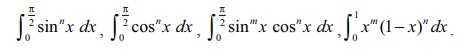Home | | Maths 12th Std | Reduction Formulae

# Reduction Formulae

Certain definite integrals can be evaluated by an index-reduction method.

Reduction Formulae

Certain definite integrals can be evaluated by an index-reduction method. In this section, we obtain the values of the following definite integrals:We also obtain the value of the improper integral Ōł×Ōł½0 eŌłÆx xn dx .

The method of obtaining a reduction formula has the following steps:

Step 1 : Identify an index (positive integer) n in the integral.

Step 2 : Put the integral as In.

Step 3 : Applying integration by parts, obtain the equation for In in terms of InŌłÆ1 or InŌłÆ2.

The resulting equation is called the reduction formula for In.

We list below a few reduction formulae without proof:Using the reduction formulas I and II, we obtain the following result (stated without proofs):By applying the reduction formula III iteratively, we get the following results (stated without proof):

(i) If n is even and m is even,(ii) If n is odd and m is any positive integer (even or odd), thenNote

If one of m and n is odd, then it is convenient to get the power of cos x as odd. For instance, if m is odd and n is even, thenExample 9.39

Find the values of the following:By applying the reduction formula III iteratively, we get the following results (stated without proof):Tags : Applications of Integration | Mathematics , 12th Maths : UNIT 9 : Applications of Integration
Study Material, Lecturing Notes, Assignment, Reference, Wiki description explanation, brief detail
12th Maths : UNIT 9 : Applications of Integration : Reduction Formulae | Applications of Integration | Mathematics